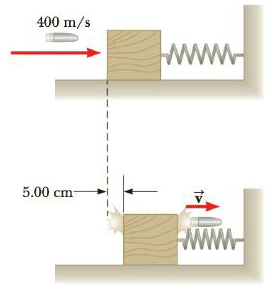Chapter 13, Problem 68AP

Chapter
Section
Textbook Problem

A 5.00-g bullet moving with an initial speed of 400. m/s is fired into and passes through a 1.00-kg block, as in Figure P13.68. The block, initially at rest on a frictionless horizontal surface, is connected to a spring with a spring constant of 900. N/m. If the block moves 5.00 cm to the right after impact, find (a) the speed at which the bullet emerges from the block and (b) the mechanical energy lost in the collision.Figure P13.68

(a)

To determine
The speed at which the bullet emerges from the block.

Explanation

Given info: The mass of the bullet is 5.00g . The initial speed of the bullet is 400ms-1 . The spring constant of the spring which is connected to the block is 900Nm-1 . The mass of the block is 1kg . After the impact the block moves 5.00cm to the right.

The conservation of mechanical energy,

(KE+PEg+PEs)f=(KE+PEg+PEs)i (1)

• KE is the kinetic energy
• PEg is the gravitational potential energy
• PEs is the elastic potential energy

Consider initial state to be just after the collision and the final state to when the block comes to rest. Then (1) will turn out to be,

0+0+12kx2=12MV2+0+012kx2=12MV2

• k is the spring constant of the spring
• x is the displacement of the spring from the equilibrium position
• M is the mass of the block
• V is the velocity of the block just after the collision

Solving for V ,

V=kx2M

Substituting k=900Nm-1 , x=5.00cm and M=1kg ,

V=(900Nm-1)(5.00cm)21kg=(900Nm-1)(0

(b)

To determine
The lost in mechanical energy in the collision.

Still sussing out bartleby?

Check out a sample textbook solution.

See a sample solution

The Solution to Your Study Problems

Bartleby provides explanations to thousands of textbook problems written by our experts, many with advanced degrees!

Get Started